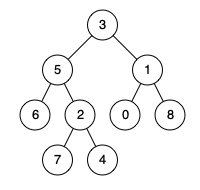# 236. Lowest Common Ancestor of a Binary

Description：
Given a binary tree, find the lowest common ancestor (LCA) of two given nodes in the tree.
According to the definition of LCA on Wikipedia: “The lowest common ancestor is defined between two nodes p and q as the lowest node in T that has both p and q as descendants (where we allow a node to be a descendant of itself).”
Note:
You may assume that all inputs are consist of lowercase letters a-z.
All inputs are guaranteed to be non-empty strings.
Difficulty：Medium
Given the following binary tree: root = [3,5,1,6,2,0,8,null,null,7,4]Example:

Input: root = [3,5,1,6,2,0,8,null,null,7,4], p = 5, q = 4
Output: 5
Explanation: The LCA of nodes 5 and 4 is 5, since a node can be a descendant of itself according to the LCA definition.


#### 方法：递归

• Time complexity : O ( n ) O\left (n \right )
• Space complexity : O ( n ) O\left (n \right )
思路：
递归到最底层，如果路径中有要找的节点，返回true，如果一个节点的左右或者自己得到两个true，那么此节点就是最近祖先。
/**
* Definition for a binary tree node.
* struct TreeNode {
*     int val;
*     TreeNode *left;
*     TreeNode *right;
*     TreeNode(int x) : val(x), left(NULL), right(NULL) {}
* };
*/
class Solution {
public:
TreeNode* res;
TreeNode* lowestCommonAncestor(TreeNode* root, TreeNode* p, TreeNode* q) {
helper(root, p, q);
return res;
}

bool helper(TreeNode* root, TreeNode* p, TreeNode* q){
if(!root) return false;

int left = helper(root->left, p, q) ? 1 : 0;
int right = helper(root->right, p, q) ? 1 : 0;
int self = (root == p || root == q) ? 1 : 0;
if(left+self+right >= 2) res = root;
return left+self+right > 0 ? true : false;
}
};

08-25295709-07568
04-061173
04-2559
10-2822
05-07185
09-10336
12-1966
01-0261
02-0634